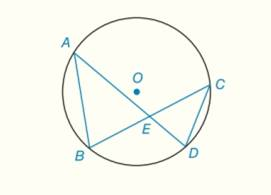Chapter 6.CT, Problem 16CTElementary Geometry For College St...

7th Edition
Alexander + 2 others
ISBN: 9781337614085

Solutions

Chapter
SectionElementary Geometry For College St...

7th Edition
Alexander + 2 others
ISBN: 9781337614085
Textbook Problem

Provide the missing statements and reasons in the following proof.Given: In ⊙ O , chords A D ¯   and B C ¯ intersects at E .Prove: A E C E = B E D EPROOF Statements Reasons 1.                                                               _ 1.                                                               _ 2. ∠ A E B ≅ ∠ D E C 2.                                                               _ 3. ∠ B ≅ ∠ D 3.If two inscribed angles intercept the same arc, these angles are congruent 4. Δ A B E ∼ Δ C D E 4.                                                               _ 5.                                                               _ 5.CSSTP

To determine

To prove:

AECE=BEDE by providing the missing statement in,

 PROOF Statements Reasons 1.                                _ 1.                                _ 2. ∠AEB≅∠DEC 2.                                _ 3. ∠B≅∠D 3.If two inscribed angles intercept the same arc, these angles are congruent 4. ΔABE∼ΔCDE 4.                                _ 5.                                _ 5.CSSTP
Explanation

Given:

In O, chords AD¯ and BC¯ intersects at E.

 PROOF Statements Reasons 1.                                _ 1.                                _ 2. ∠AEB≅∠DEC 2.                                _ 3. ∠B≅∠D 3

Still sussing out bartleby?

Check out a sample textbook solution.

See a sample solution

The Solution to Your Study Problems

Bartleby provides explanations to thousands of textbook problems written by our experts, many with advanced degrees!

Get Started

11. Is if?

Mathematical Applications for the Management, Life, and Social Sciences

In Exercises 516, evaluate the given quantity. log1,000

Finite Mathematics and Applied Calculus (MindTap Course List)

j × (−k) = i −i j + k −j − k

Study Guide for Stewart's Multivariable Calculus, 8th

In Exercises 9-14, state whether the statements are true or false. a. 0b. 0=

Finite Mathematics for the Managerial, Life, and Social Sciences